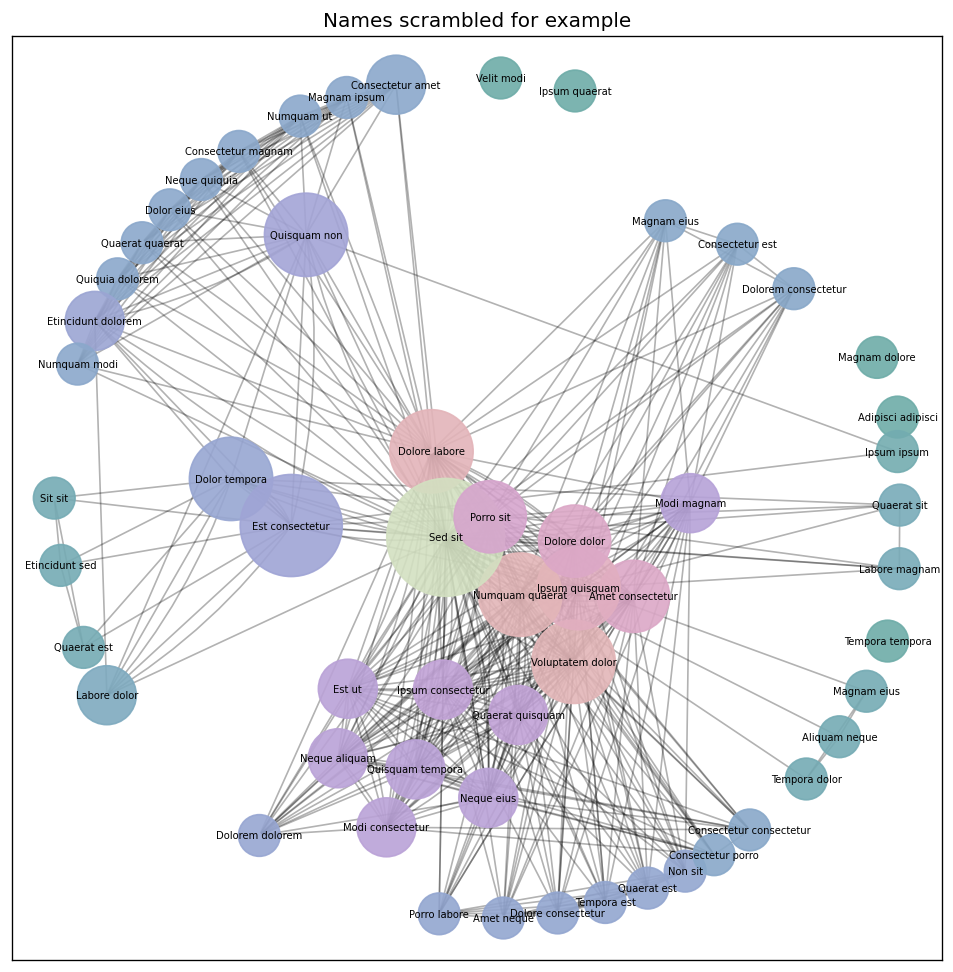# Zoviz¶

Zoviz is an unaffiliated library for accessing and visualizing Zotero data.

Gitlab project: https://gitlab.com/jlogan03/zoviz (includes example.ipynb notebook)

## Examples¶

### Visualizing a graph of collaboration¶

```with zoviz.DB() as db:  # Zotero database is discovered automatically
g = db.build_creator_graph(collection="example")
zoviz.draw_community_graph(g, edge_color='k')
```### Querying the database¶

```with zoviz.DB() as db:
example_query = \
"""
SELECT value FROM itemDataValues WHERE valueID IN (
SELECT valueID FROM itemData WHERE itemID IN (
SELECT itemID FROM items WHERE itemTypeID IN (
SELECT itemTypeID FROM itemTypes WHERE typeName IN
( 'journalArticle' ) ) ) )
"""

df = db.query_df(example_query)
print(df["value"])
```

returns

```Cooling topologies for superconducting power systems: II. Long-distance electric transmission
```

### Accessing tables¶

Because Zotero databases can be assumed to be “small” in computational terms, it’s usually safe to access tables directly. Whenever a table is accessed directly, if it isn’t in memory, it will be queried at that time.

```# Three ways to get the itemData table as a pandas DataFrame
with zoviz.DB() as db:
# The shortest way
itemData = db.itemData
# The short way
itemData = db["itemData"]
# The long way
itemData = db.query_df("SELECT * FROM itemData")
```